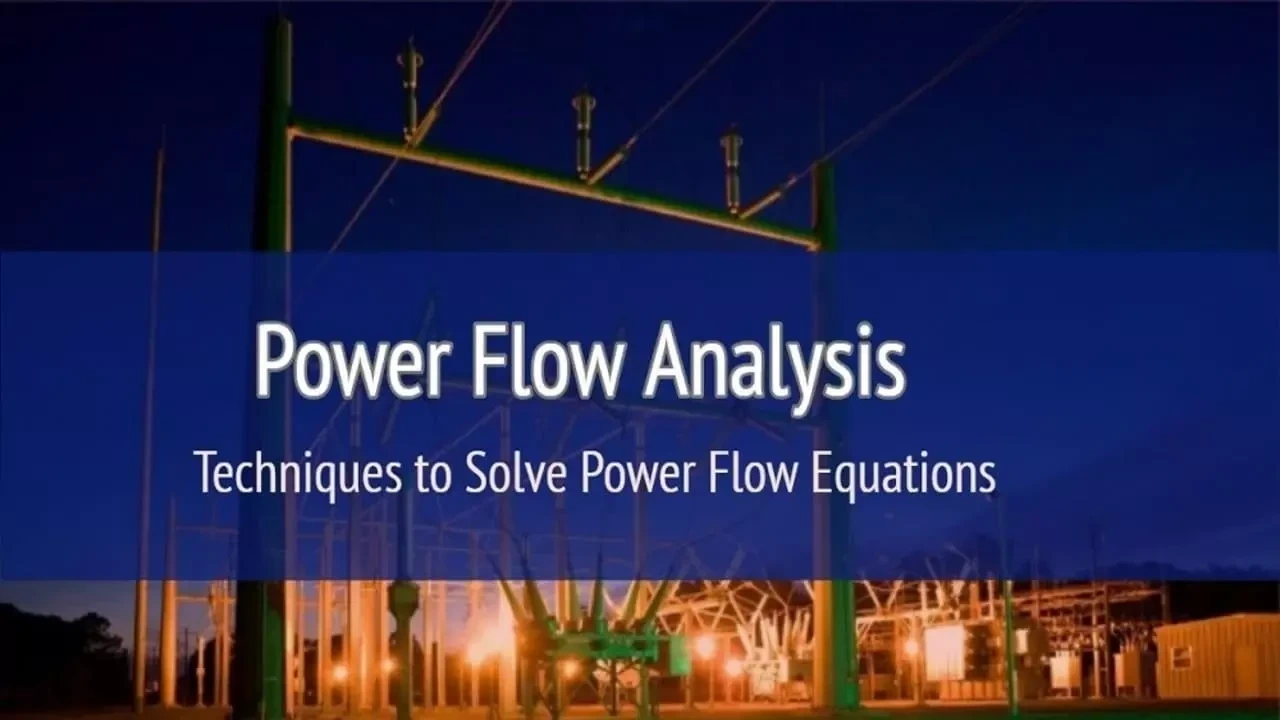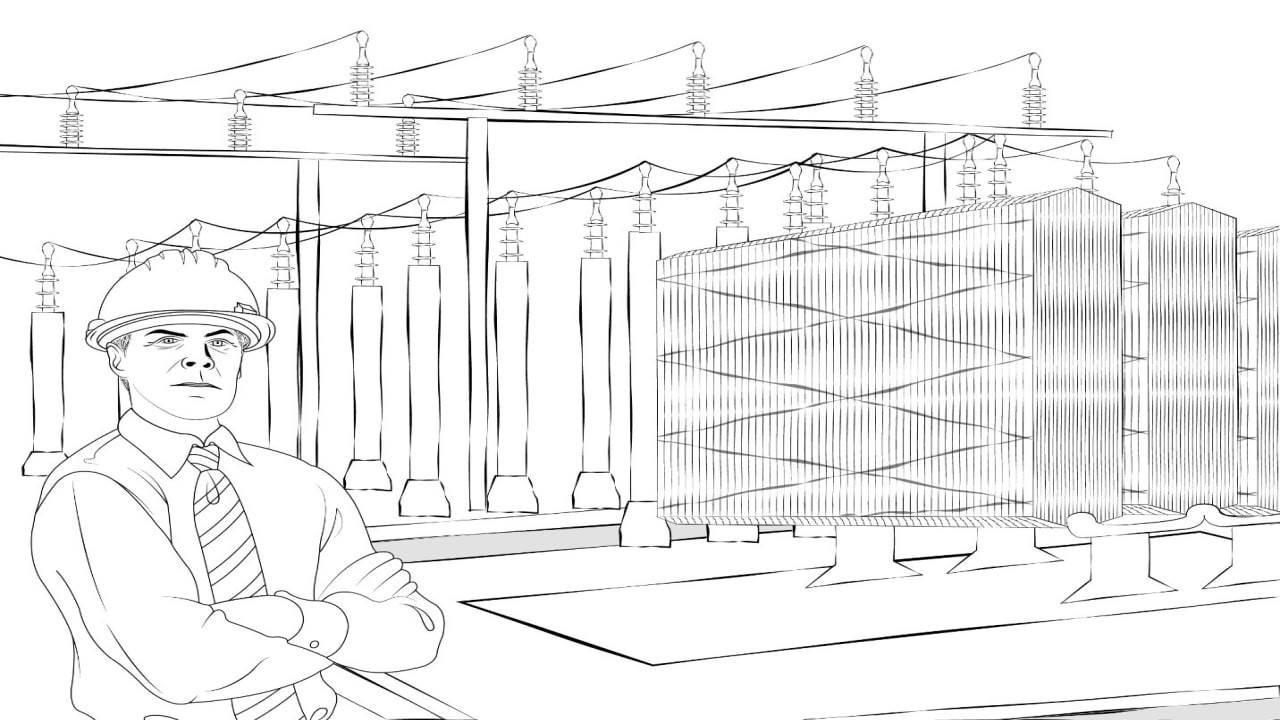(206) 687-4009 | [email protected]

# Power Flow AnalysisLast updated: Sep 18, 2020

Power flow, or load flow, is widely used in power system operation and planning. The power flow model of a power system is built using the relevant network, load, and generation data. Power engineers are required to plan, design, and maintain the power system to operate reliably and within safe limits. Numerous power flow studies are required to ensure that power is adequately delivered at all times despite normal load fluctuations and undesirable events such as contingencies. Daily fluctuations in the power system operations cause power flow mismatches at busbars. As a result, the busbars voltage magnitude and angle adjust instantly until an equilibrium is reached between the load and the transmitted power. This new equilibrium point can also be obtained from simulation using power flow methods.

## Different Techniques to Solve Power Flow Equations

Outputs of the power flow model include voltages at different buses, line flows in the network, and system losses. These outputs are obtained by solving nodal power balance equations. Since these equations are nonlinear, the following iterative techniques are commonly used to solve this problem.

Power flow studies consist in determining the voltage magnitude and angle at each busbar until there is an equilibrium in active and reactive power at which point power mismatches are insignificant. Calculating the final values of the busbars voltage is not always straightforward especially for heavily loaded and complex systems.

The starting point of solving power flow problems is to identify the known and unknown variables in the system. Based on these variables, buses are classified into three types: slack, generation, and load buses as shown in the following table:Types of Bus Variables

Real power, P

Reactive Power, Q

Voltage Magnitude |V| Voltage Angle
Slack Bus Unknown Unknown Known

known

Generator Bus (PV) known Unknown

Known

Unknown

Known Known

Unknown

Unknown

Hello there! On a related topic, we previously wrote a blog about Power Factor - An Overview. If this peaks your interest, check it out and let us know what you think

### Newton-Raphson Power Flow Method

Newton Raphson method is a numerical technique for solving non-linear equations. It is often classified as iterative root finding scheme. The reason it is called root finding is, it is geared towards solving equations like f(x)=0 (or f(x)=0). The solution to such an equation, call it x* (or x*), is clearly a root of the function f(x) (or f(x)). The first order Newton-Raphson (NR) method is considered as the state of the art for power flow calculations. This method has been widely used in industry applications.

It is iterative because it requires a series of successive approximations to the solutions. The procedure is generally as follows. First, guess a solution. Unless we are very fortunate, the guess will be, of course, wrong. So, we determine an update to the "old" solution that moves to a "new" solution with the intention that the "new" solution is closer to the correct solution than was the "old" solution.

A key aspect to this type of procedure is the way we obtain the update. If we can guarantee that the update is always improving the solution, such that the "new" solution is in fact always closer to the correct solution than the "old" solution, then such a procedure can be guaranteed to work if only we are willing to compute enough updates, i.e., if only we are willing to iterate enough times.

• Fast convergence if the initial guess is close to the solution
• Easy to convert to multiple dimensions, can be used to polish a root found by other methods
• Large region of convergence
• Time required for per iteration in Newton Raphson method is larger than Gauss Seidel method but the overall time for iterative process is less because of a smaller number of iterations for convergence.

• Each iteration takes much longer.
• More complicated to code, particularly when implementing sparse matrix algorithms.

### Gauss-Seidel Power Flow MethodIn Gauss Seidel method, the computations appear to be serial. Further, each component of new iteration depends upon all previously computed components. Updates cannot be done simultaneously. In addition to this, new iteration depends on the order in which equations are examined. If this ordering is changed, the components of new iteration (and not just their order) also change. These limitations persuade engineers and researchers to go for Newton Raphson method.

• Gauss Seidel method is easy to program.
• Each iteration is relatively fast (computational order is proportional to number of branches and number of buses in the system).
• Acquires less memory space than NR method.

• Tends to converge relatively slowly, although this can be improved with acceleration.
• Has tendency to miss solutions, particularly on large systems.
• Tends to diverge on cases with negative branch reactance (common with compensated lines).
• Need to program using complex numbers.

### Fast Decoupled Power Flow MethodIn high voltage transmission systems, the voltage angles between adjacent buses are relatively small. In addition, X/R ratio is high. These two properties result in a strong coupling between real power and voltage angle and between reactive power and voltage magnitude. In contrary, the coupling between real power and voltage magnitude, as well as reactive power and voltage angle, is weak. Considering adjacent buses, real power flows from the bus with a higher voltage angle to the bus with a lower voltage angle. Similarly, reactive power flows from the bus with a higher voltage magnitude to the bus with a lower voltage magnitude.

Fast-decoupled power flow technique includes two steps:

• decoupling real and reactive power calculations.
• obtaining of the Jacobian matrix elements directly from the Y-bus.

As the size of matrix becomes very large for a big bus system so for faster and less memory allocation, we prefer decoupled load flow where we take P independent of V and Q Independent of δ, and thus those Jacobian elements are taken as zero.

• The convergence is faster than other methods.
• The memory requirement is very less than the other methods like as Newton Raphson method, Gauss-Seidel method, etc.
• This method is less complicated than other methods, therefore it is more easy method to calculate the power flow.
• The number of iteration and size of equation used is less.

• Take more iterations though time needs for each iteration is less than NR method
• The accuracy of the fast-decoupled load flow is mainly dependent on three factors:
• System size and structure;
• Convergence tolerances; and
Particularly in large systems with heavy loading the relatively small error in the state variables may cause larger errors in real and reactive power flow, but these errors are small in comparison with the line ratings. The accuracy of the solution is a controllable parameter and it can be improved by using a smaller convergence tolerance.

### Comparison of the Load Flow Methods

Load Flow Method Speed Accuracy Solution Robustness
Gauss-Seidel Very Slow Approximate Robust
Newton Raphson Slow Accurate Robust
Fast Decoupled Fast Accurate Less Robust

### Industry Application of Power Flow Methods

Commercial power systems are complicated. It is not possible to analyze power flow through hand calculations. Physical models of power systems were analyzed through network analyzers in laboratories between 1929 and early 1960. Afterwards, invention of digital computers replaced the analog methods with numerical methods. Initially, linear methods were proposed to analyze power flow analysis. Among these, Cramer's method, Gauss elimination and LU factorization are notable. However, these methods cannot handle complex, nonlinear and big power systems. Therefore, iterative techniques i.e., Gauss Seidel method, Newton Raphson method are developed to solve complex power systems.There are a number of very high-quality commercial power flow programs on the market today, some of which include those developed by the Electric Power Research Institute (EPRI), Power Technologies Incorporated (PTI), Operation Technology, Inc., and EDSA. Most of today's commercial software packages are menu-driven from a Windows environment. Few of the commonly used software are discussed briefly as below:

• ETAP software is used for simulation because of its extension of real time intelligent power management systems for monitoring, controlling, automating and optimizing power systems. It is a high impact software used for power flow analysis is generation, transmission and distribution systems of electric power engineering.
• PowerWorld Simulator is a software package designed to simulate high voltage power systems up to 100,000 buses. PowerWorld uses the Newton-Raphson iteration method, which provides an efficient and accurate solution. It also computes the Jacobian (Admittance Matrix), so that the one-line diagram characteristics can be ported to other analysis programs.
• CYME is a software which main objective is to analyze the steady-state performance of the power system under various operating conditions. It is the basic analysis tool for the planning, design and operation of any electrical power systems, be they distribution, industrial or transmission networks. The Power Flow module utilizes state-of-the-art sparse matrix/vector methods and multiple solution algorithms.
Check out Power System Analysis Fundamentals Course in which we briefly discussed "Power Flow Equations".
Close